# Perform Corner Detection by Using Neighborhood Processing Subsystem Blocks

This example shows how to detect corners in an image by using Neighborhood Processing Subsystem blocks. Use corner detection to identify features and objects in an image.

### Inspect Model

1. Open the model.

```mdl = 'CornerDetectionNeighborhoodExample'; open_system(mdl);```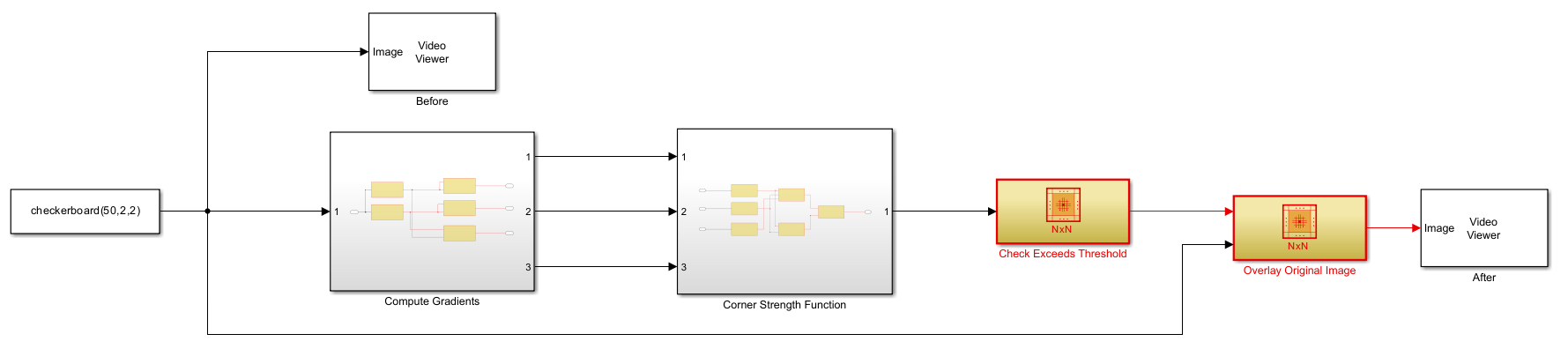The model performs Harris corner detection. Harris corner detection calculates a value $\mathit{R}$ for each pixel. The $\mathit{R}$ value computes the rate of change of brightness in multiple directions around a pixel and reflects whether the pixel represents an edge, corner, or flat region in the image.

• A flat region has low rates of change in all directions and yields an $\mathit{R}$ value near zero.

• An edge has a high rate of change in only one direction and yields a negative $\mathit{R}$ value.

• A corner has high rates of change in all directions and yields a positive $\mathit{R}$ value.

The model marks any pixel with a positive $\mathit{R}$ values as a corner, and uses two Video Viewer blocks from Computer Vision Toolbox to display the image with and without the corner markings.

2. Return to the model root and open the `Compute Gradients` subsystem.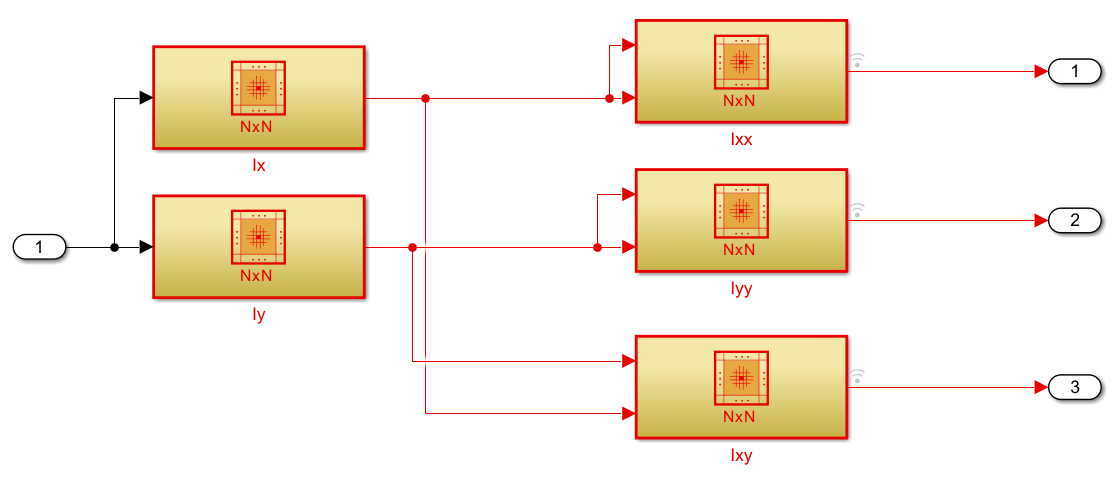The `Ix` and `Iy` Neighborhood Processing Subsystem blocks compute the gradients of brightness with respect to the horizontal and vertical directions respectively, ${\mathit{I}}_{\mathit{x}}$ and ${\mathit{I}}_{\mathit{y}}$. The `Ixx`, `Iyy`, and `Ixy` Neighborhood Processing Subsystem blocks each contain a Product block and compute the products of the brightness gradients, ${{\mathit{I}}_{\mathit{x}}}^{2}$, ${{\mathit{I}}_{\mathit{y}}}^{2}$, and ${\mathit{I}}_{\mathit{x}}{\mathit{I}}_{\mathit{y}}$.

3. Return to the model root and open the `Corner Strength Function` subsystem.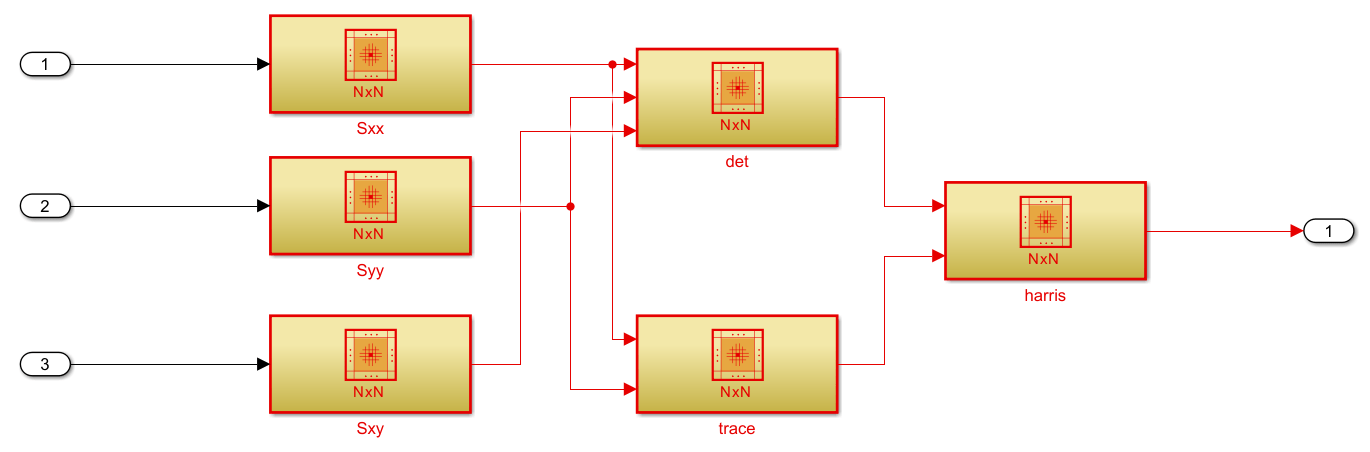The subsystem computes $\mathit{R}$ for each pixel. Consider the change function $\mathit{E}\left(\mathit{u},\mathit{v}\right)$:

$\mathit{E}\left(\mathit{u},\mathit{v}\right)=\sum _{\mathit{x},\mathit{y}}\mathit{w}\left(\mathit{x},\mathit{y}\right){\left[\mathit{I}\left(\mathit{x}+\mathit{u},\mathit{y}+\mathit{v}\right)-\mathit{I}\left(\mathit{x},\mathit{y}\right)\right]}^{2}$.

For a neighborhood $\mathit{w}\left(\mathit{x},\mathit{y}\right)$, $\mathit{E}\left(\mathit{u},\mathit{v}\right)$ calculates the weighted sum of squared differences between the brightness in the neighborhood, $\mathit{I}\left(\mathit{x},\mathit{y}\right)$, and the brightness in a neighborhood of the same size shifted by $\left(\mathit{u},\mathit{v}\right)$, $\mathit{I}\left(\mathit{x}+\mathit{u},\mathit{y}+\mathit{v}\right)$. Applying Taylor expansion yields this approximation:

$\mathit{E}\left(\mathit{u},\mathit{v}\right)\approx \left[\begin{array}{cc}\mathit{u}& \mathit{v}\end{array}\right]\left(\sum _{}\left[\begin{array}{cc}{{\mathit{I}}_{\mathit{x}}}^{2}& {\mathit{I}}_{\mathit{x}}{\mathit{I}}_{\mathit{y}}\\ {\mathit{I}}_{\mathit{x}}{\mathit{I}}_{\mathit{y}}& {{\mathit{I}}_{\mathit{y}}}^{2}\end{array}\right]\right)\left[\begin{array}{c}\mathit{u}\\ \mathit{v}\end{array}\right]$.

The `Sxx`, `Syy`, and `Sxy` Neighborhood Processing Subsystem blocks each contain a Sum of Elements block and compute the sums of the brightness gradient products ${\sum }_{}{{\mathit{I}}_{\mathit{x}}}^{2}$, ${\sum }_{}{{\mathit{I}}_{\mathit{y}}}^{2}$, and ${\sum }_{}{\mathit{I}}_{\mathit{x}}{\mathit{I}}_{\mathit{y}}$.

Consider the matrix $\mathit{M}$:

$\mathit{M}=\sum _{}\mathit{w}\left(\mathit{x},\mathit{y}\right)\left[\begin{array}{cc}{{\mathit{I}}_{\mathit{x}}}^{2}& {\mathit{I}}_{\mathit{x}}{\mathit{I}}_{\mathit{y}}\\ {\mathit{I}}_{\mathit{x}}{\mathit{I}}_{\mathit{y}}& {{\mathit{I}}_{\mathit{y}}}^{2}\end{array}\right]$.

Computing the eigenvalues of $\mathit{M}$ yields the rates of change in brightness around the neighborhood $\mathit{w}\left(\mathit{x},\mathit{y}\right)$. Computing eigenvalues directly is computationally expensive, so the subsystem calculates the determinant and trace of $\mathit{M}$, which relate to the eigenvalues ${\lambda }_{1}$ and ${\lambda }_{2}$ in these ways:

• $\mathrm{det}\left(\mathit{M}\right)={\lambda }_{1}{\lambda }_{2}$

• $\mathrm{trace}\left(\mathit{M}\right)={\lambda }_{1}+{\lambda }_{2}$

4. Open the `det` subsystem to see how the subsystem calculates the determinant of $\mathit{M}$, $\mathrm{det}\left(\mathit{M}\right)$.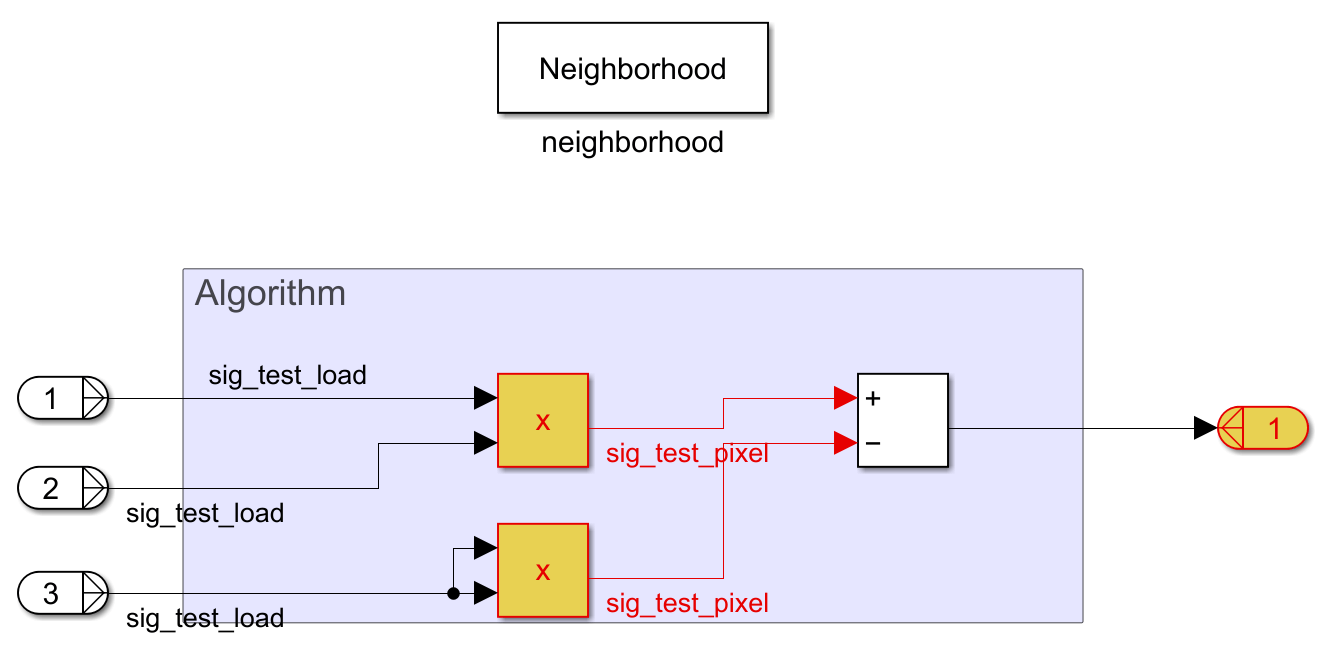5. Return to the `Corner Strength Function` subsystem and open the `trace` subsystem to see how the subsystem calculates the trace of $\mathit{M}$, $\mathrm{trace}\left(\mathit{M}\right)$.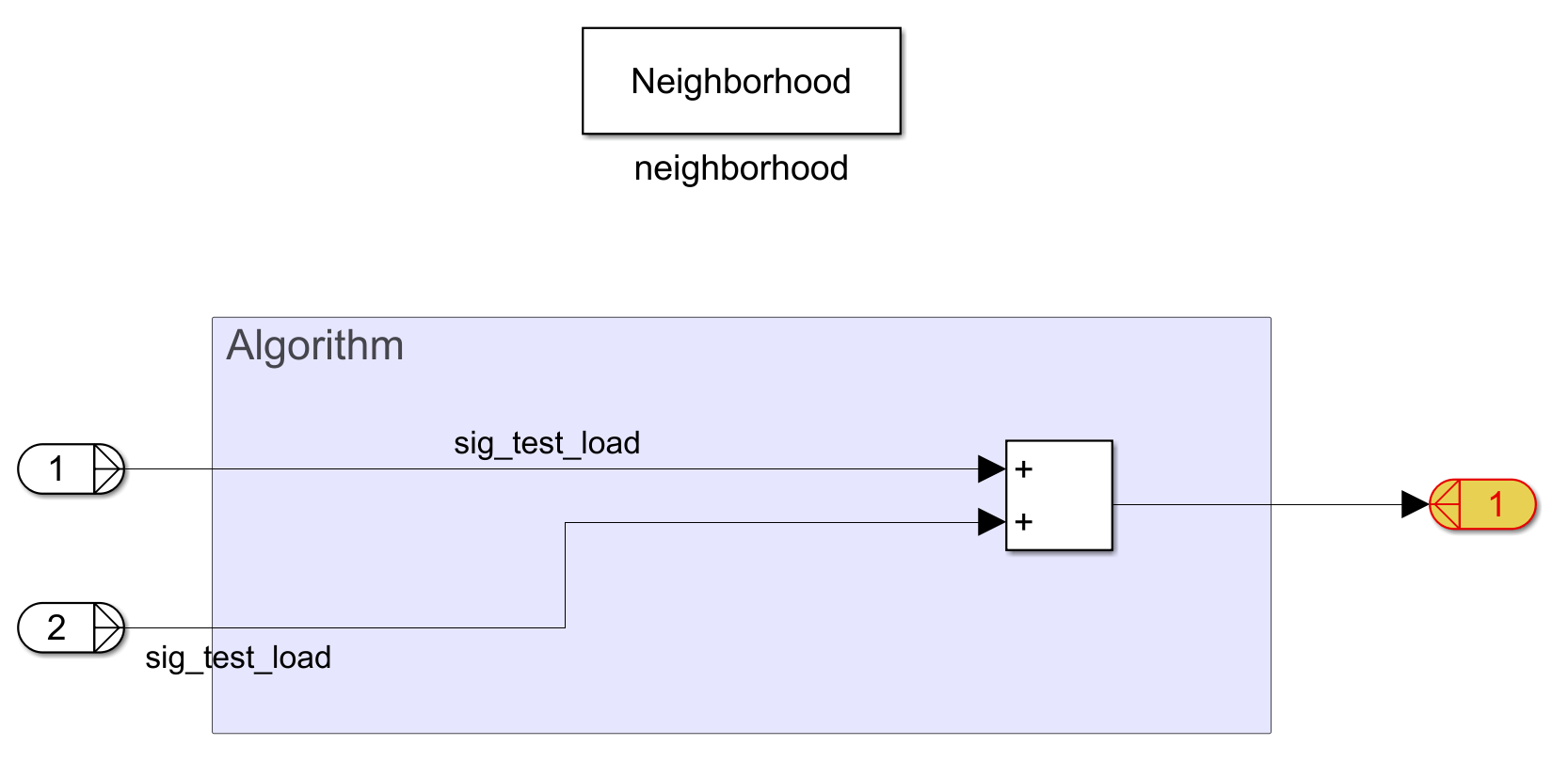6. Return to the `Corner Strength Function` subsystem and open the `harris` subsystem to see how the subsystem calculates $\mathit{R}$.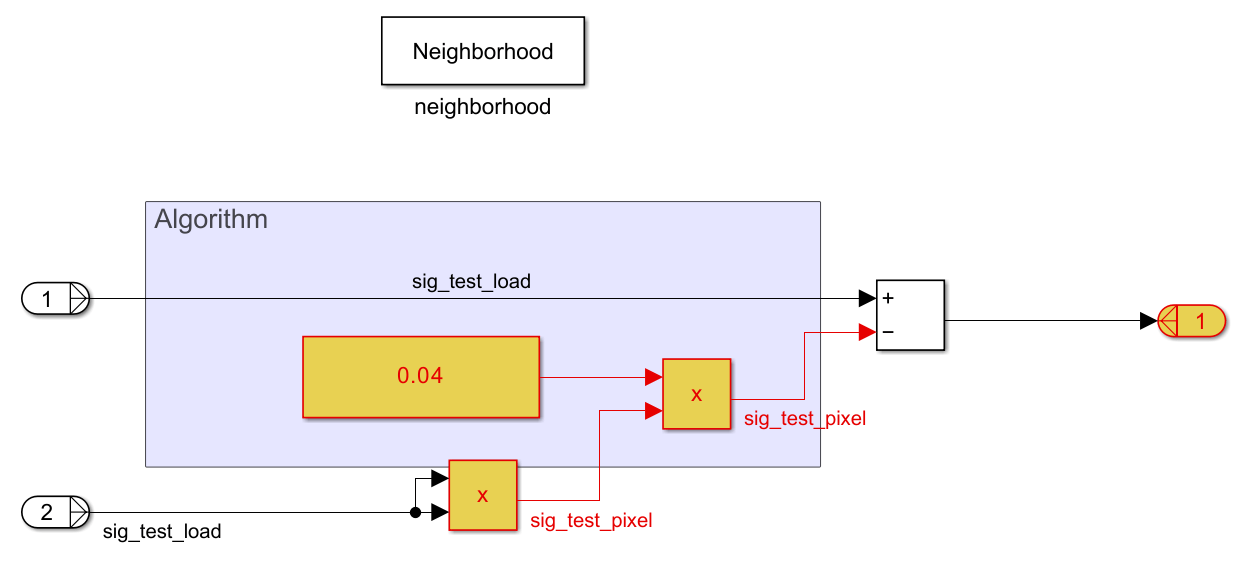The `harris` subsystem calculates $\mathit{R}$ using this equation:

$\mathit{R}=\mathrm{det}\left(\mathit{M}\right)-0.04×{\mathrm{trace}\left(\mathit{M}\right)}^{2}$.

7. Return to the model root and open the `Check Exceeds Threshold` subsystem.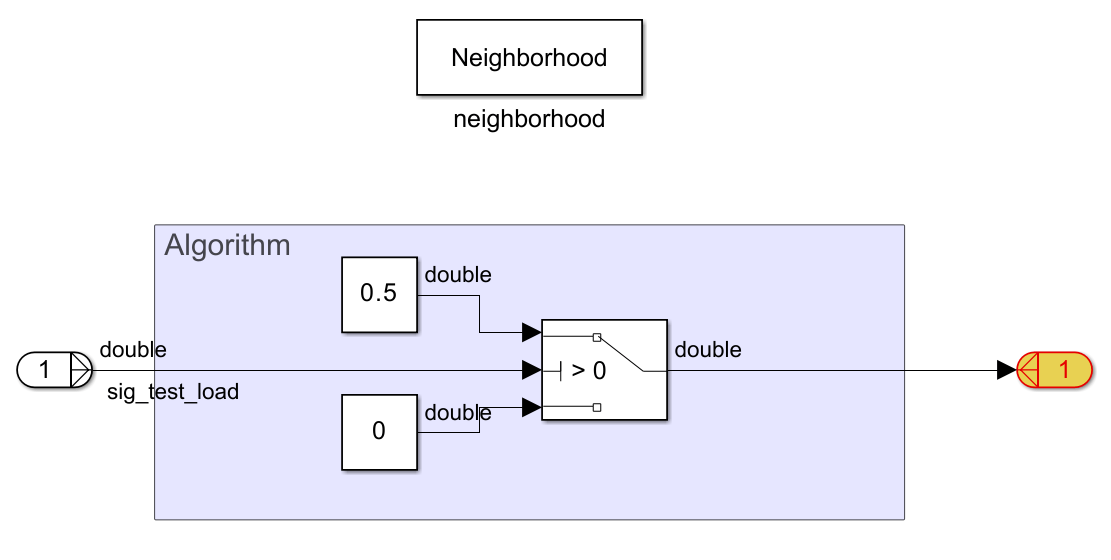The subsystem uses a Switch block to identify pixels with positive $\mathit{R}$ values, which represent corners. The neighborhood subsystem returns the value `0.5` for these pixels, which creates a moderate shade of gray in the output image.

8. Return to the model root and open the `Overlay Original Image` subsystem.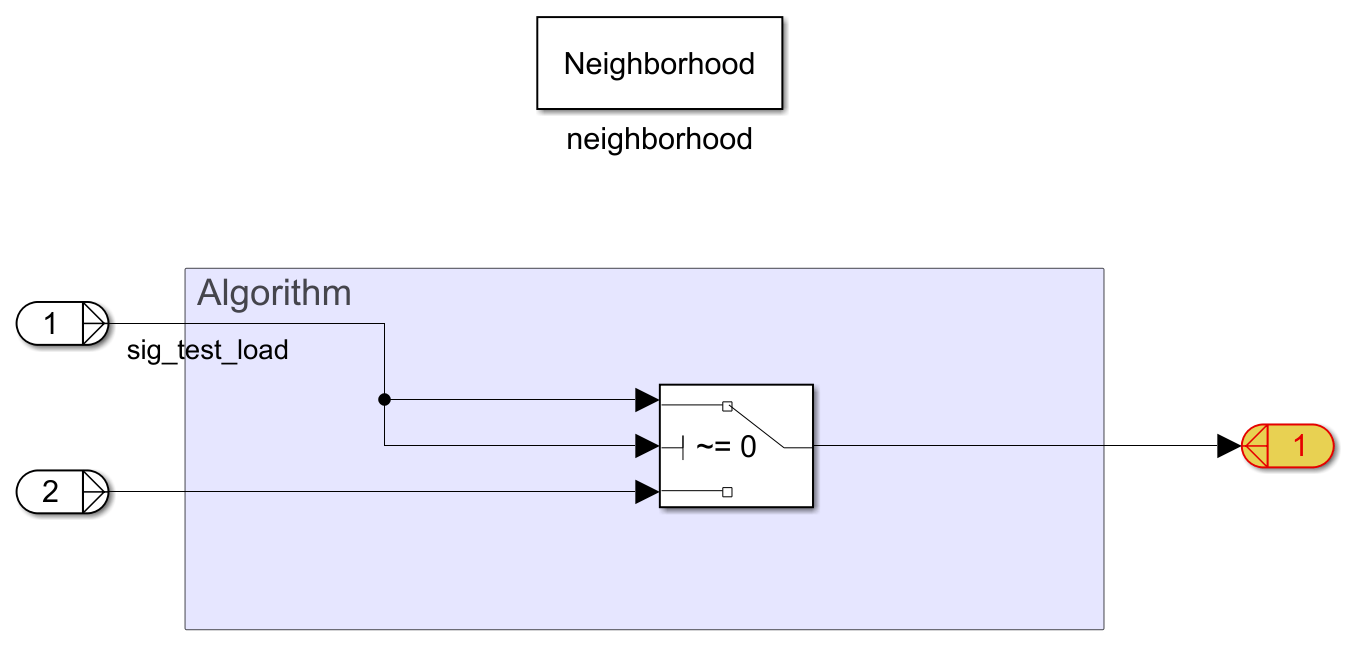The subsystem uses a Switch block to overlay the corner markings and the original image.

### Simulate and View Results

Simulate the model.

`simout = evalc('sim(mdl)');`

The `Before` Video Viewer block displays the original checkerboard image.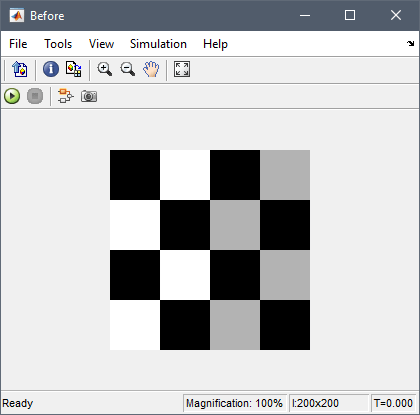The `After` Video Viewer block displays the checkerboard image with gray dots marking the corners.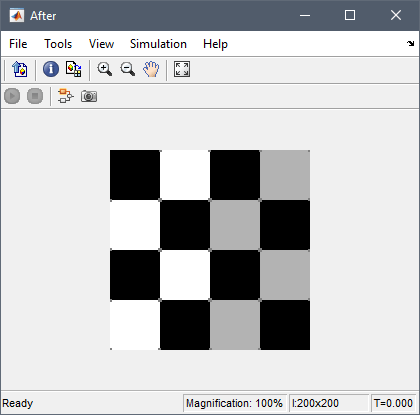The upper left and lower right corners do not have markers because the Neighborhood Processing Subsystem blocks in the model use the `Constant` padding option with a padding value of `0`. The Padding option block parameter controls how the Neighborhood Processing Subsystem block treats pixels in neighborhoods that extend beyond the input image. The parameters in the model configure the Neighborhood Processing Subsystem blocks to use the value `0` outside the input image, which treats the image as if it is surrounded by black pixels. Thus, the model recognizes only the white and gray corners of the image as corners.